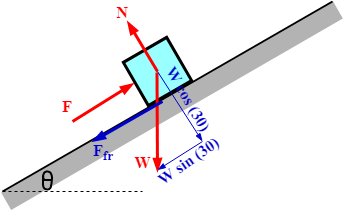# How can I find the power in Watts, supplied by a man at a time t, given a mass in kg, a force in...

## Question:

How can I find the power in Watts, supplied by a man at a time t, given a mass in kg, a force in Newtons, time in seconds, and the coefficient of kinematic friction, pushing a block up an incline at an angle of 30 degrees, initially the crate is at rest?

## Work done by a Force:

If a force is applied on a body, the body tends to move. The work done by the force can be determined by the product of the applied force and the distance moved by the body. If the body moves on an inclined plane then the components of the forces must carefully be considered while resolving the forces.

Draw the free-body diagram.Resolve the forces perpendicular to the plane.

{eq}N=W\cos \theta \\ N=mg\cos \theta {/eq}

Resolve the forces parallel to the plane.

{eq}F-W\sin \theta -\mu N=0\\ =W\sin \theta +\mu N\\ =mg\sin \theta +\mu mg\cos \theta \\ F=mg(\sin \theta +\mu \cos \theta ) {/eq}

Find the power or the rate of work done by the force.

{eq}P=\dot W=F\times d\\ P=mgd(\sin \theta +\mu \cos \theta )\\ \bf \color {navy} {P=mgd[\sin (30^\circ ) +\mu \cos 30^\circ]} {/eq}

Knowing the values of 'm', 'd' and {eq}'\mu ' {/eq}, the power in Watt can be determined.